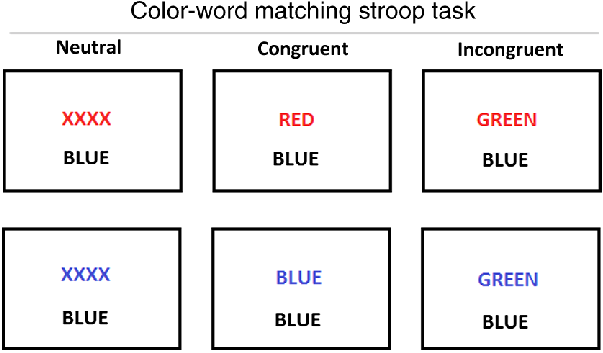# Python exercises: Plotting¶

from jupyterquiz import display_quiz


During the exercises in the dataframes module you learned how to import a dataset, and how to look at the dataframe from multiple angles. In the python lessons for this module you learned that you can use matplotlib in three different ways. From “quick-and-rigid” to “slow-but-flexible”, these are: (1) Seaborn plotting (2) Pyplot procedural plotting (3) Object-oriented plotting

For the exercises below we will load in the same dataframe as you used in dataframe_exercises. This dataframe contains data for a Stroop task. You can try out the Stroop task for yourself here. In the dataframe, there is “congruency” column which defines which condition the trial (row) was:

• “inc” stands for “incongruent” (word and color do not match)

• “con” stands for “congruent” (word and color match)

• “neutral” stands for trials where there was no word, but only color

See below for examples of each stimuli:display_quiz("questions/question_1.json")


## Exercise 1. Seaborn plotting¶

Now, using seaborn, make a bar plot of the response time of each value in ‘congruency’. Give the plot a title. Which value has the highest response times?

import pandas as pd
import seaborn as sns
import matplotlib.pyplot as plt



## Exercise 2: Matplotlib Procedural Plotting¶

Now, make the same plot using the matplotlib procedural plotting approach (don’t forget to include the title as well).

# your answer here


## Exercise 3: Matplotlib Object-Oriented Plotting¶

Yes, you guessed it: now plot the same plot using the object-oriented approach (again, title!).

# your answer here